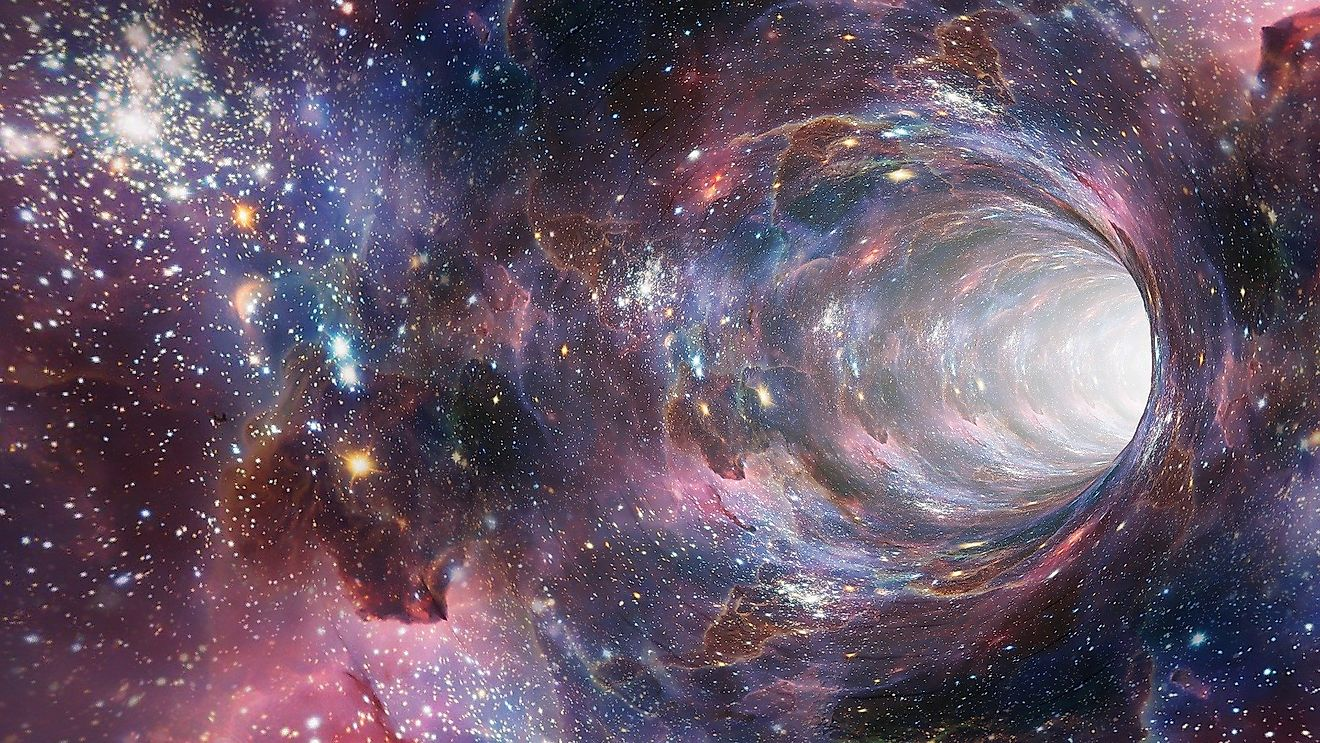# What Is A Singularity?In the world of physics, a singularity is a concept that shifts the laws of physics as we know them. Image by Genty from Pixabay
• Space-time is a crucial concept in theoretical physics, and it views both space and time as a single entity.
• The Chandrasekhar limit is a critical mass threshold of a star, and it is 1,39 solar masses.
• Three main types of singularities are Curvature, Conical, and Naked singularities.

In the world of physics, a singularity is a concept that shifts the laws of physics as we know them. The theories on singularity came about when people first discovered black holes. The unusual nature of black holes has made scientists ask the question - what lies beyond?

Singularity, in this context, serves as a theoretical framework to explain the Big Bang, and gravity becomes the focus of the exploration.

## Gravitational Singularity

Physicists have proposed the idea of the so-called gravitational singularity. From this type of standpoint, a gravitational singularity is an occurrence or an object where common laws of physics do not work. This type of singularity is a specific point in space-time, a construct that views the notions of time and space like they are almost glued together.

## Space-Time

This gravitational singularity is a hard one to measure, at least in a traditional sense of the word. In fact, a space-time singularity becomes virtually independent of the coordinate system, or space, where it is observed. The data that is measured becomes, in a way, infinite. Because of this, a singularity creates a system where time and space no longer affect each other and practically become one thing. That is why the phrase ‘’space-time’’ is so essential because the two entities stop having self-governing properties.

## Black Holes And Einstein

It does not come as a shock, how Albert Einstein, arguably one of the greatest minds of the 20th century, is the person responsible for this hard-grasping concept. After Einstein came out with his Theory of General Relativity, it was possible to discuss singularities. It could be said that the black holes themselves were, in a way, predicted by Einstein and that he created a theoretical frame from where scientists could start to unveil the mysteries that lie beyond the event horizon.

The theory of how black holes, and therefore singularities, are possible, is not that hard to conceptualize. When a particular star becomes to approach a certain point of its mass, it creates a force of gravity that is so strong that the star collapses into itself. This breaking point is called the Chandrasekhar limit. This limit is exactly 1,39 solar masses, which means mass that is 1,39 times bigger than the mass of our own Sun. When a star collapses, nothing, not even light, can escape it. When that happens, we are talking about something called the event horizon

## Curvature, Conical, And Naked Singularities

There are two distinct types of singularities that exist if the event horizon covers them. The first one is known as Curvature singularity. It got its name because of what happens inside the black hole. At the very center, a black hole holds up enormous amounts of mass. Because of this, gravity becomes infinite, which leads to the, also infinite, curving of space-time.

The other type that goes by Conical singularity happens when the singularity reaches a point where all the variables are finite. In this scenario, the space-time is not infinite, but it looks more like a cone, with the Conical singularity at its very top.

Another type of singularity is the one that does not depend on it being covered up by the event horizon. In this case, we are talking about Naked singularity. The Naked singularity does not stay hidden behind the event horizon. In theory, this type of singularity existed before the Big Bang.

Share